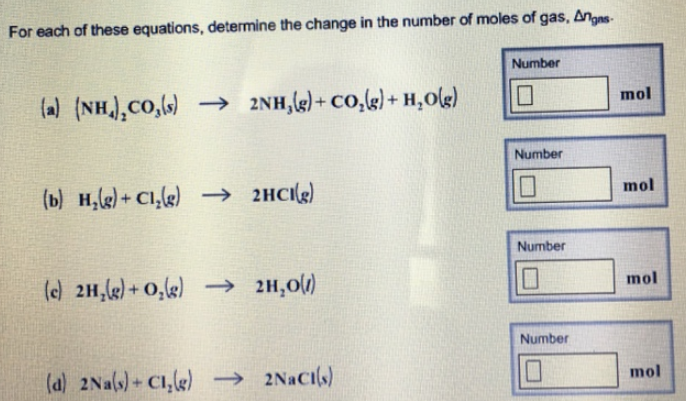# Problem: For each of these equations, determine the change in the number of moles of gas, Δngas. (a) (NH4)2CO3 (s) → 2NH3 (g) + CO2 (g) + H2O (g) (b) H2 (g) + Cl2 (g) → 2HCl (g) (c) 2H2 (g) + O2 (g) → 2 H2O(l) (d) 2Na (s) + Cl2 (g) → 2NaCl (s)

###### FREE Expert Solution
85% (36 ratings)###### Problem Details

For each of these equations, determine the change in the number of moles of gas, Δngas

(a) (NH4)2CO3 (s) → 2NH3 (g) + CO2 (g) + H2O (g)

(b) H2 (g) + Cl2 (g) → 2HCl (g)

(c) 2H2 (g) + O2 (g) → 2 H2O(l)

(d) 2Na (s) + Cl2 (g) → 2NaCl (s)